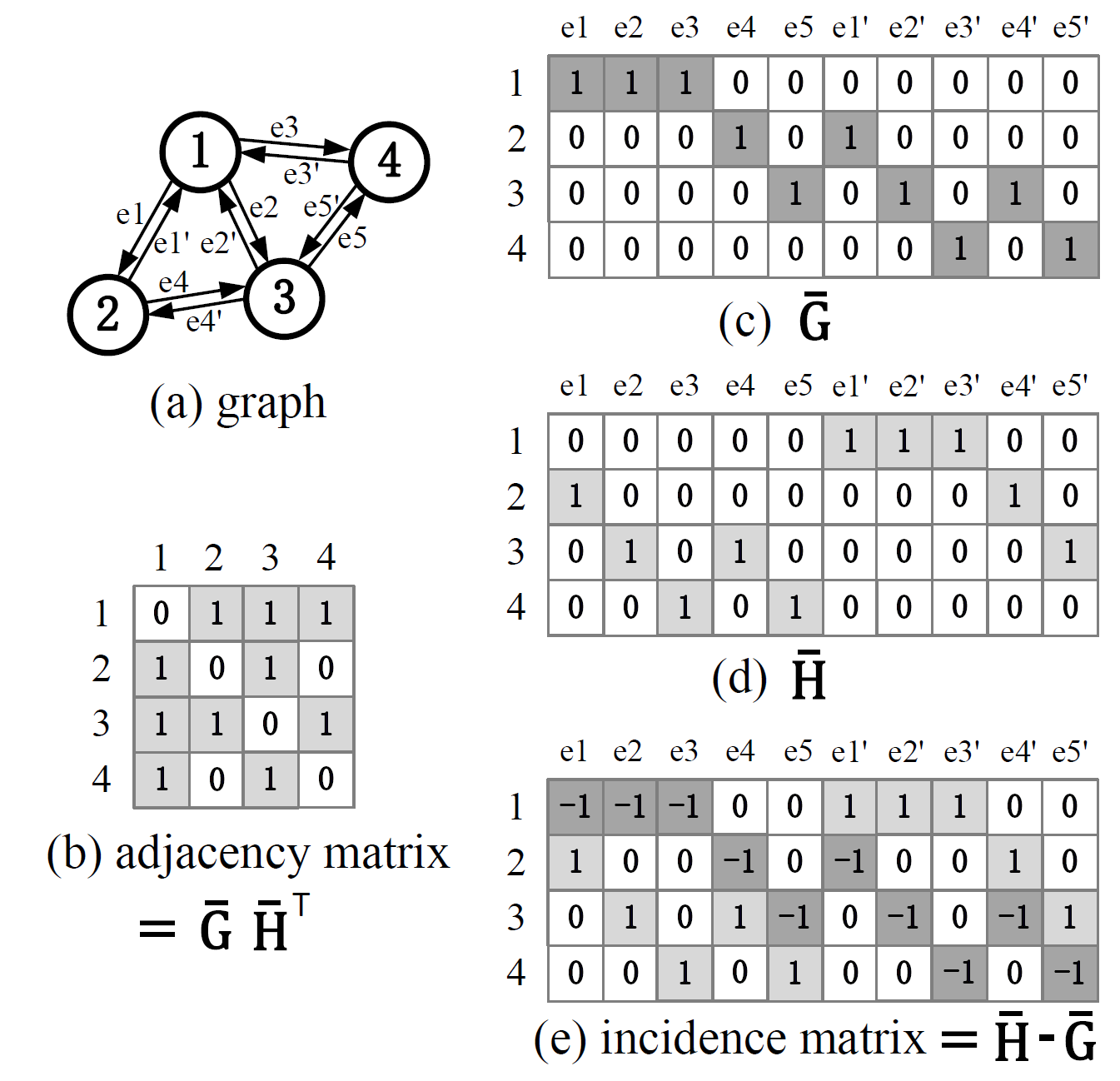# src.build_graphs.build_graphs¶

src.build_graphs.build_graphs(P_np: numpy.ndarray, n: int, n_pad: Optional[int] = None, edge_pad: Optional[int] = None, stg: str = 'fc', sym: bool = True, thre: int = 0) Tuple[numpy.ndarray, numpy.ndarray, numpy.ndarray, int][source]

Build graph matrix $$\mathbf G, \mathbf H$$ from point set $$\mathbf P$$. This function supports only cpu operations in numpy. $$\mathbf G, \mathbf H$$ are constructed from adjacency matrix $$\mathbf A$$: $$\mathbf A = \mathbf G \cdot \mathbf H^\top$$

Parameters
• P_np$$(n\times 2)$$ point set containing point coordinates

• n – number of exact points in the point set

• stg – strategy to build graphs. Options: fc, near, tri

• sym – True for a symmetric adjacency, False for half adjacency (A contains only the upper half)

• thre – The threshold value of ‘near’ strategy

Returns

$$A$$, $$G$$, $$H$$, edge_num

The possible options for stg:

'fc'(default): construct a fully-connected graph
'near': construct a fully-connected graph, but edges longer than thre are removed
'tri': apply Delaunay triangulation


An illustration of $$\mathbf G, \mathbf H$$ with their connections to the graph, the adjacency matrix, the incident matrix is